﻿ Magnetization of IPM MotorExamples | Product | Murata Software Co., Ltd.Example9Magnetization of IPM Motor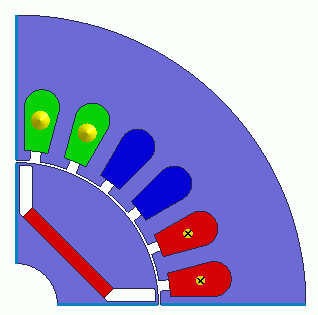General

• The magnetization of the IPM motor (interior permanent magnet motor) is analyzed.
The analysis takes the following two steps.

1. Magnetization Analysis
A magnetization current is applied to the magnet.

2. Analysis Using Magnetization Results
The magnetization results give by step 1 are set to the magnet to analyze the motor torque.

• The magnetization ratio, the magnetization distribution, the torque, and the magnetic flux density distribution are solved.

• The homogenizing method is applied to simulate the layer structure of the electromagnetic plates of steel for the core.

• Unless specified in the list below, the default conditions will be applied.

• In this example, a quarter period symmetric model is used for faster calculation.

Show Results

 Item Setting Analysis Space 2D Thickness in Depth Direction 60[mm] Unit mm Solver Magnetic Analysis [Luvens] Analysis Type Transient Analysis Options [Partial Model (Symmetric Model) Setting] Select Partial Model. Division number: 4 Select Convert the result to the full model for output

Set the Mesh Tab as follows.

 Tab Setting Item Setting Mesh Set the general mesh size automatically: Deselect General Mesh size: 1[mm] Automatic Ambient Air Creation Select Create ambient air automatically Ambient Air Scale: 1.2

The transient analysis tab is set as follows.

• The intensity of the magnetic field is obtained for each timestep. At some timestep, the intensity drops. The magnetization field is determined by the intensity of the magnetic field at a timestep just before it drops.
The timestep setting does not affect the result as the constant current is used in this example.
The same result will be obtained if the timestep setting is switched to [steady-state analysis].

• In order to take the induced current into account, input waveform has to be of the magnetization current instead of the constant current.

Tab

Setting Item

Setting

Transient Analysis

Timestep

Manual

Table

 Number Calculation steps Output steps Timestep [s] 1 1 1 1

Model

A rotor core and magnet are placed in the center. A stator and coils are placed around them.

The motor has 4 poles and 24 slots.

The magnetization current is set to the coils I1,I2,I3, and I4.

This is a 2D model analysis.

By utilizing the symmetry, the model is quarter period symmetric.

Rotation period boundary (symmetric) is set.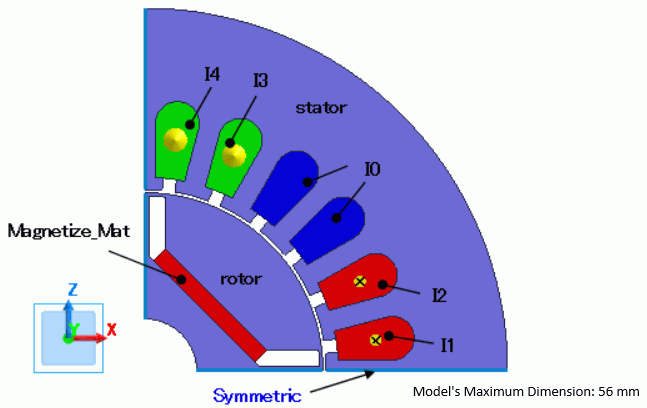Body Attributes and Materials

 Body Number/Type Body Attribute Name Material Name 12/Sheet Mag Magnetize_Mat 11/Sheet Rotor 35JN270_JFE Steel* 28/Sheet Stator 35JN270_JFE Steel* 30/Sheet U1+ 008_Cu * 31/Sheet U1+ 008_Cu * 32/Sheet W1- 008_Cu * 33/Sheet W1- 008_Cu * 34/Sheet V1+ 008_Cu * 35/Sheet V1+ 008_Cu *

* Available from the material DB

The body attribute is set up as follows.

The magnetization current is set to the coils I1,I2,I3, and I4.

A variable [current] is used for easy modification.

For the core, the homogenizing method is selected to simulate the layered steel plates.

 Body Attribute Name Tab Setting Mag Direction Vector: X=1, Y=0, Z=1 Current Click Yes for induced current setting. Rotor Layer Select Take layer into account Space: 97[%] Layer direction vector: X=0, Y=1, Z=0 Stator Layer Select Take layer into account Space: 97[%] Layer direction vector: X=0, Y=1, Z=0 I1, I2 Current Waveform: Constant Current value: current (using variable) Turns: 35[Turns] Direction: +Y direction I3, I4 Current Waveform: Constant Current value: current (using variable) Turns: 35[Turns] Direction: – Y direction I0 Current Waveform: Constant Current: 0[A]

The material properties are set as follows.

The magnetization characteristics are defined. See Permeability tab for details of the parameters of the magnetization characteristics.

Material Name

Tab

Properties

Magnetize_Mat

Permeability

Material type: Magnetizing material

Initial Magnetization Characteristics

B-H Curve Table

 Magnetic Field [A/m] Magnetic Flux Density [T] 0 0 76000 0.18 152000 0.36 228000 0.55 304000 0.75 380000 0.98 456000 1.20 532000 1.40 608000 1.58 684000 1.75 760000 1.90 836000 2.05 912000 2.20 988000 2.50 1064000 2.75 1140000 2.89 1216000 3.00 1292000 3.10 1444000 3.30 2010000 4.00

Definition Type of Magnetization Rate: Normalize with coercivity

Magnetization Ratio Table

 Magnetization Field [A/m] Magnetization Ratio Recoil Permeability 0 0 1.8 152000 0.01 1.77 456000 0.1 1.72 684000 0.2 1.58 836000 0.1 1.33 988000 0.6 1.26 1064000 0.8 1.18 1292000 0.91 1.13 1672000 0.97 1.08 2000000 1 1.05

Coercivity at magnetization ratio of 1.0: 980000[A/m]

Boundary Condition

Half period symmetric boundary is set.

 Boundary Condition Name/Topology Tab Boundary Condition Type Setting Symmetric Symmetry/Continuity Periodic Rotation Period (Half Period)

Results

There are two steps of analysis as follows.

1. Magnetization Analysis
A magnetization current is applied to the magnet.

2. Use Magnetization Result
The magnetization results give by step 1 are set to the magnet to analyze the motor torque.

The results of 1 are shown below. (The results of the analysis model [Magnetization analysis].)

The magnetization distribution with the magnetization current (variable “current”) of 100 to 1900 [A] is shown below.

The magnetization is almost fully achieved at 1900 [A].

 100[A] 700[A] 1300[A] 1900[A]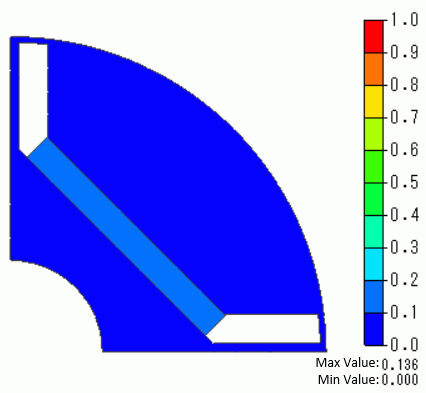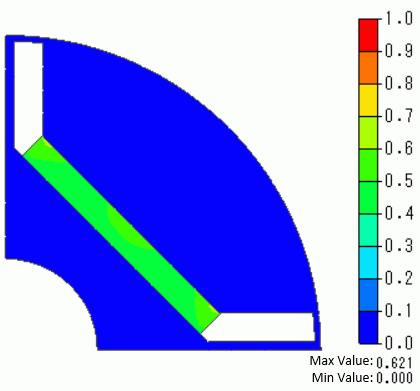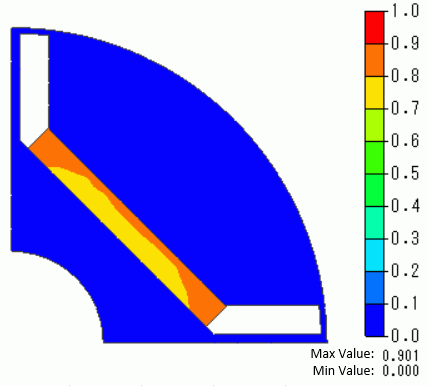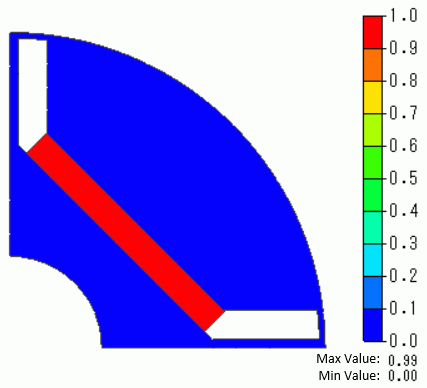The diagram below shows the magnetization distribution.

 100[A] 700[A] 1300[A] 1900[A]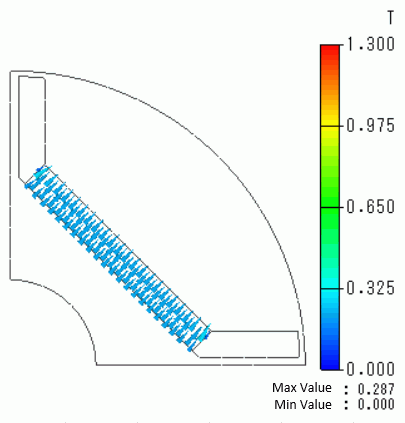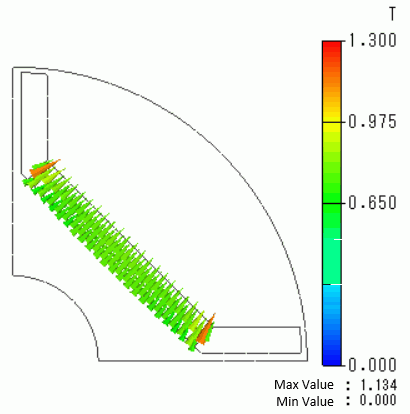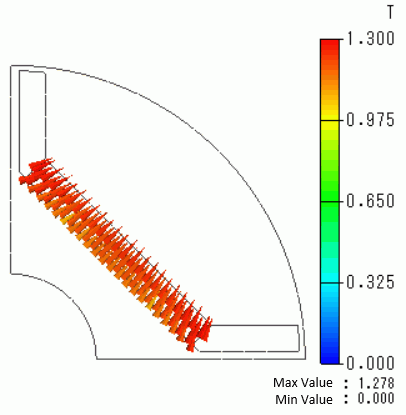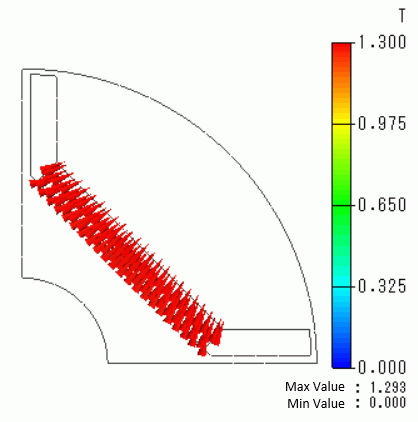The analysis result of step 2 is shown below. (The results of the analysis model [Torque analysis (use magnetization results].)

In this analysis model, the result of step 1 is set to the magnet.

(Material type is set to [Use Magnetization Result] on the [Permeability] tab.)

The torque wave at the magnetization current of 1300 [A] is shown below.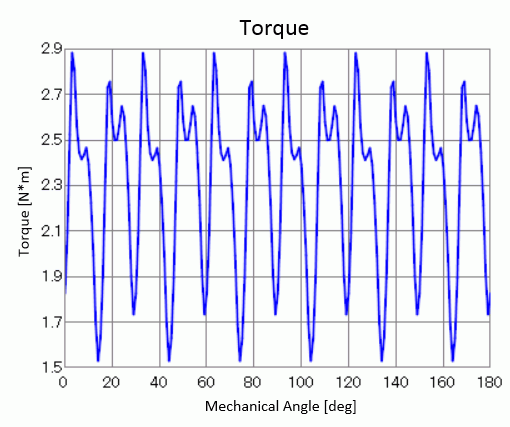The magnetic flux density at the magnetization current of 1300 [A] is shown below.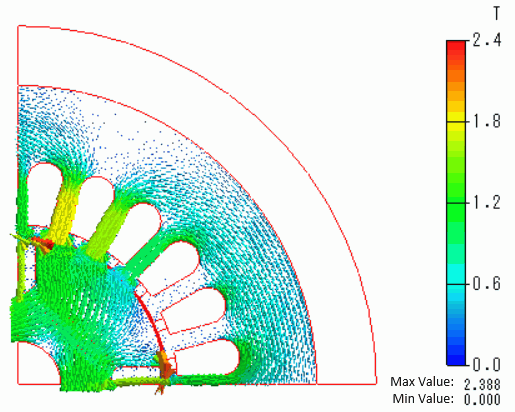﻿